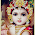# Math for Computer science Questions and Answers 151 to 160

## Math for Computer science

### Questions  151 to 160

151.
Let A = {a, b} and B be the set of all strings over A. The function g: B →Z is defined as            g(S) = the number of a’s in S, for each string S ∈B. Then g (ababb) =
 (a) 0 (b) 2 (c) 3 (d) 4 (e) 5
152.
How many different permutations can be formed out of all the letters of the word “MATHS”?
 (a) 12 (b) 24 (c) 20 (d) 120 (e) 60
153.
How many different permutations can be formed out of the letters of the word “MISSISSIPPI”?
 (a) (11!) / (7!) (b) (11!) / (3!) (c) (11!) / (2!. 4!. 4!) (d) (11!) / (4!) (e) (11!)/(4!. 3!).
154.
How many groups of 4 people can be formed from 6 people?
 (a) 15 (b) 30 (c) 60 (d) 120 (e) 45
155.
How many ways can 5 persons arrange themselves around a circular table?
 (a) 120 (b) 24 (c) 5 (d) 720 (e) 60
156.
Ten balls numbered 0, 1, 2, ….., 9 are put in a box and two balls are taken at random without replacement. The  probability that both numbers are odd is equal to
 (a) (b) (c) (d) (e) 1.
157.
A box contains 4 red balls and 2 white balls. Another box contains 5 red balls and 3 white balls. Abox is selected at random and then a ball is drawn at random. The probability that the ball is white is equal to
 (a) (b) (c) (d) (e) 0.
158.
If A and B be two events with P(A) = , P(B) =   and P(A È B) = , then P(A/B) =
 (a) (b) (c) 0 (d) (e) .
159.
If A and B be two events with P(A) = , P(B) =  and P(A Ç B) = , then P(Ac Ç Bc) =
 (a) (b) (c) (d) (e) .
160.
It is given that p → q, q → r and r → s are true. Which of the following must be necessarily true?
 (a) q → p (b) p → r (c) s → r (d) p → s (e) q → s.

#### Answers

 151 Answer : (b) Reason  :       by the definition of function the number of a’s are in the given string is 2 152 Answer : (d) Reason  :       by the definition of permutations the number of ways are  (5!) 153 Answer : (c) Reason  :       by the definition of permutations with indistinguishable objects  the number of ways are  (11!) / (2! 4! 4!) 154 Answer : (a) Reason  :       4 people has to be selected from 6 people which can be done in C(6,4) ways = 15 ways 155 Answer : (b) Reason  :       by circular permutation the  number of ways are (5-1)! = 4! = 2456 156 Answer : (d) Reason  :       The no. of ways choosing 2 balls is C (52,2 ).  The first odd number can be drawn in C(5,2 ) ways and that of second odd number in C(4,2 ) ways . the probability is given by  4/9 ( after simplification of  [C(5,2 )x C(4,2 )] / C (52,2 )  ) 157 Answer : (d) Reason  :       The probability of choosing a box is ½.  The white ball choosing from 1 box is 2/6   and  The white ball choosing from 2 box is 3/6 so the required probability is 1/6+3/16 158 Answer : (c) Reason  :       P(AÇB) = 0  so  P(A/B) = 0 159 Answer : (b) Reason  :       By the  Addition theorem on probability  , P(Ac Ç Bc) = 1- P (AÈ B ) = 1 – (7/9)             =2/9 160 Answer : (d) Reason  :       By the definition of  Hypothetical syllogism

#### 1 comment :

1.incomplete answer choice